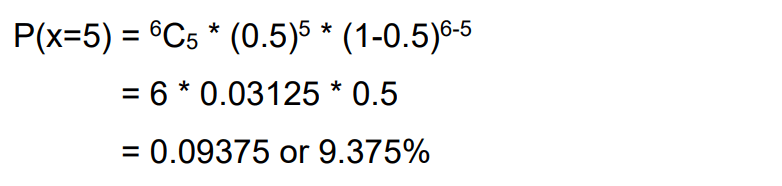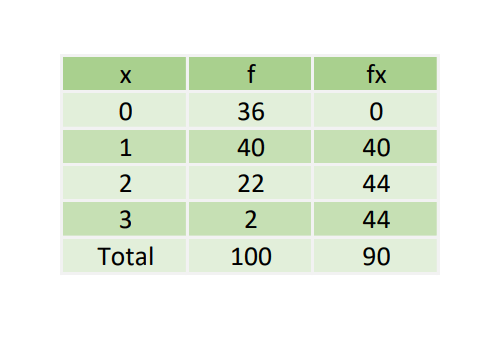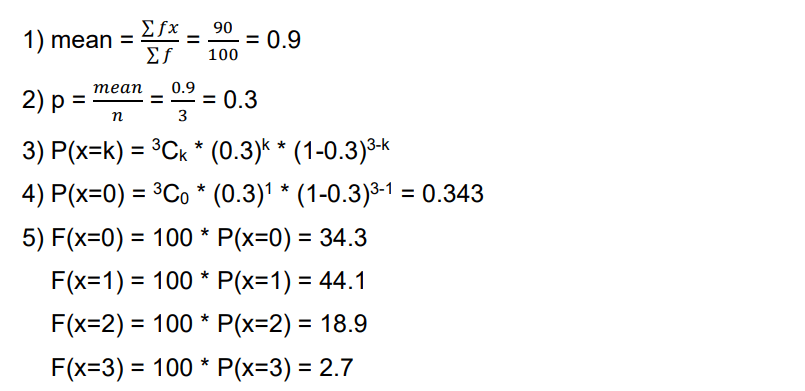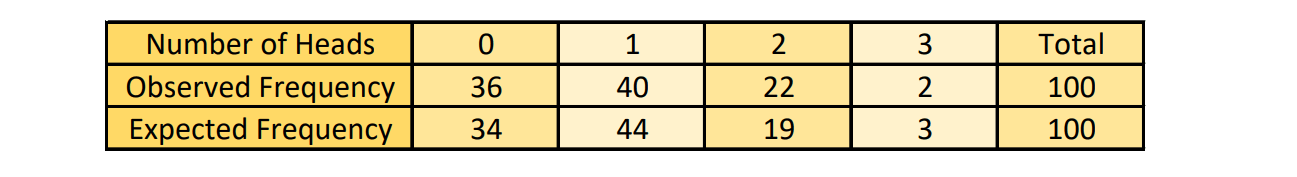## Introduction

Whenever an experiment having two possible outputs: Success or Failure, is repeated multiple times then the probability of success or failure is said to follow Binomial Distribution (‘Bi’ means two, indicating the two possible outcomes). In statistics and probability, the binomial distribution has two parameters: n (the number of times that time event or experiment has occurred), and p (the success rate when the event has occurred once).

If n = 1, then the Binomial Distribution is called the Bernoulli Trial or Bernoulli Experiment, named after Joseph Bernoulli. The Binomial Distribution is the foundation for the binomial test of statistical significance. Poisson Distribution is the limiting case of Binomial Distribution, where n is very large and p is very small.

## Binomial Distribution

Let us consider an experiment: when we toss a coin, there are two possible outcomes: either we get heads or tails. Therefore our sample space (say S) = {Heads, Tails}. If we repeat this random experiment several times, then each one is called a trial. Let us say that we want the outcome of a coin flip to be Tails. The actual outcome of each trial can be only 2 things: Either we succeed to get Tails, or we fail to get Tails. There cannot be a scenario where we get both Heads and Tails or we get neither Heads nor Tails. This is the reason why the outcome of a coin flip falls under Binomial Distribution.

#### Notation of Binomial Distribution

In order to find Binomial Distribution, we must know two important things:

• The number of trials (n)
• The success rate for each trial (p)

Therefore, the notation of Binomial Theorem is as follows:#### Properties of Binomial Distribution

There are certain properties that the Binomial Distribution must always follow:

• Each trial must be independent of the previous series of trials.
• Success and failure must be mutually exclusive, i.e., Success + Failure = 1
• There must not be an outcome other than success and failure.
• The number of trials must be finite.

#### Binomial Formula

The formula of Binomial Distribution for a probability mass function is:Here:

• n – Number of trials
• k – Number of successes in n trials
• p – Probability of success

There are certain constants in a Binomial Distribution. These are:

• Mean = np
• Variance = np*(1-p)
• Standard deviation = sqrt(Variance)

Let us assume that we are flipping a coin 6 times. We will infer success if the coin lands heads. In this case, the probability of success and failure are both 0.5 for each trial.

What can be the probability that we get heads 5 out of  6 times? We can find this using the Binomial Formula.

Here, n = 6, k = 5 and p = 0.5#### Fitting a Binomial Distribution

When a Binomial Distribution is to be fitted to a data of observations, the following procedure is adopted:

1. Find the mean of the data.
2. Find p = mean/number of data items.
3. Write the probability mass function P(x=k).
4. Put k = 0, i.e., find P(x=0)
5. Find expected frequencies F(x=k) = N * P(x=k) where N = sum of frequencies

Consider this example of fitting a Binomial Distribution:

In the data given below, a set of three identical coins are tossed 100 times, yielding the given results. Let us find the expected frequencies by fitting a Binomial Distribution.This is how the following data can be interpreted:Following the procedure mentioned above, this is how we calculate:Since frequencies cannot be non-integer in value, we will round off each of the values. Let us compare them with the observed frequencies:#### Implementation

Let us now dive into the code for the binomial formula example:

First, we define a function that finds the factorial of a given number iteratively. Then we define a function that returns the value of the Binomial Distribution. We then take some values of p, n and k to get the output.

				
fun factorial(n: Double): Double
{
var x = 1.0
var i = 2.0
while (i &lt;= n)
{
x *= i
i++
}
return x
}


				
fun binomial(p: Double, n: Double, k: Double): Double
{
return Math.pow(p,k)*Math.pow(1-p,n-k)*factorial(n)/(factorial(k)*factorial(n-k))
}



In the function above, as we pass the arguments, the combination can be written in terms of factorial for a simpler way of implementation and easy execution.

				
val p = 0.5
val n = 6.0
val k = 5.0
println(binomial(p, n, k))



Output:

				
0.09375